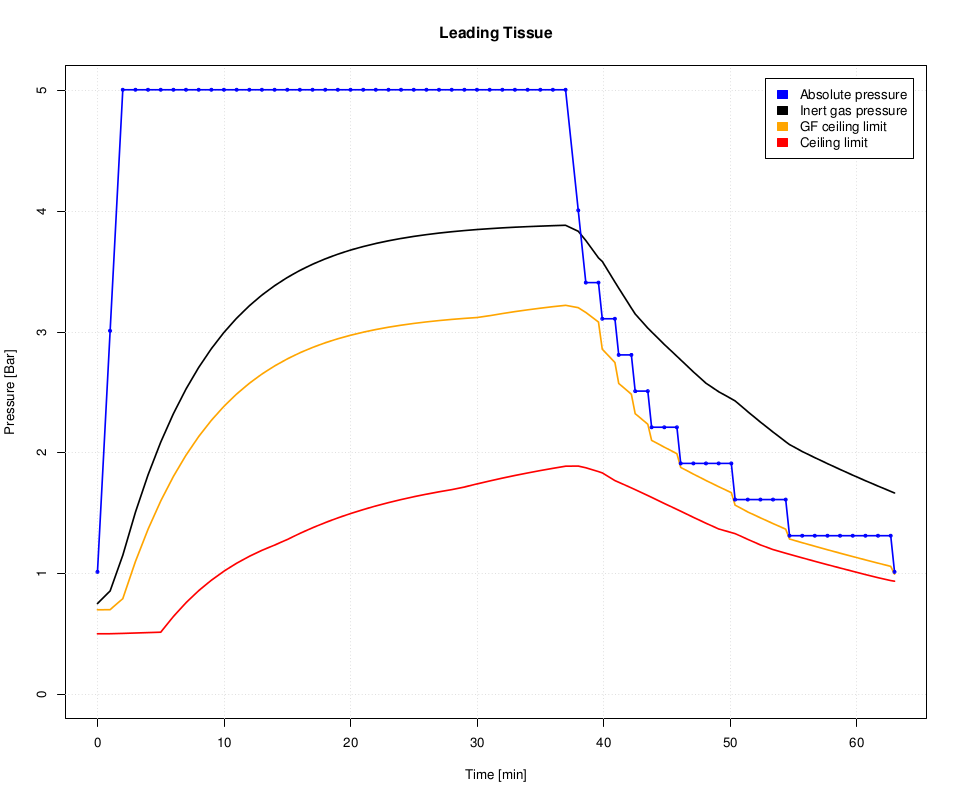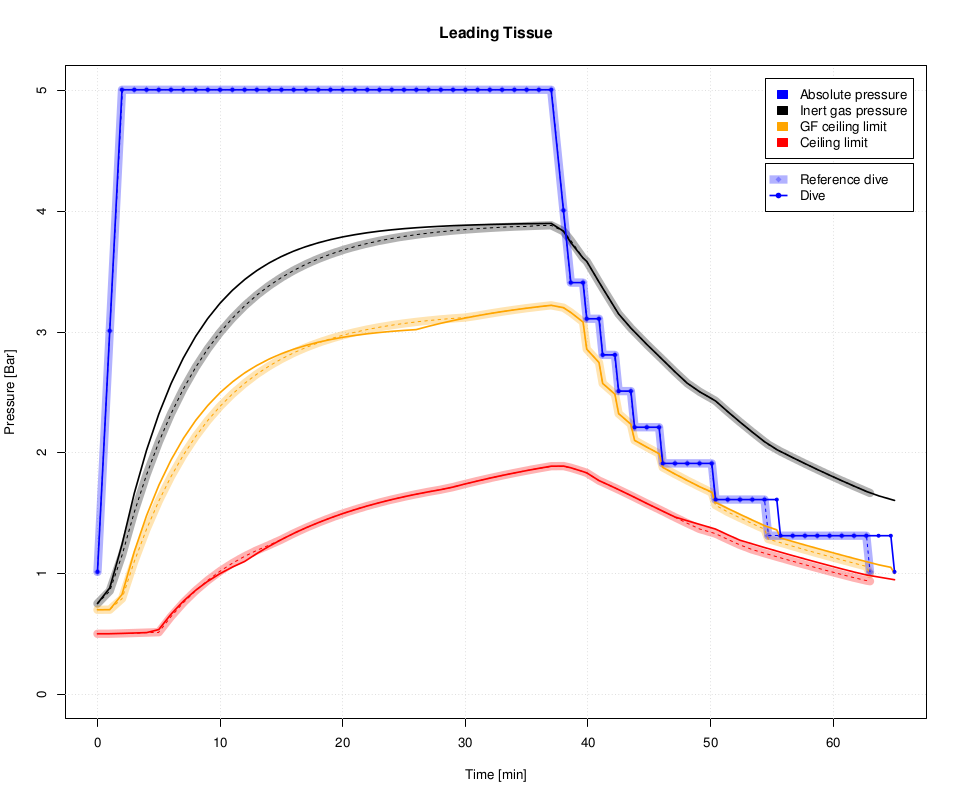# Commandline Tools¶

DecoTengu library provides two commandline applications.

The dt-lint command prints dive decompression information and allows to store dive profile steps data in a CSV file.

The dt-plot command plots dive profile steps data in the form of PDF file.

## Calculating Dive Decompression Data¶

To calculate decompression information of a dive to 40m for 35 minutes on air use the following command:

$dt-lint 40 35 Dive profile: 40m for 35min Descent rate: 20.0m/min Ascent rate: 10.0m/min GF Low: 30% GF High: 85% Surface pressure: 1013.25 millibar Gas list: o2=21% at 0m Decompression stops (ZH-L16B-GF): 21m 1min 18m 1min 15m 2min 12m 5min 9m 7min 6m 14min 3m 25min ------------- Sum: 55min  The dive profile steps data can be saved using -f option. An example of saving the data into dive.csv file with dive steps calculated every minute: $ dt-lint -f dive.csv -t 1 -gl 20 -gh 90 -l '21,0@0 50,0@21 100,0@6' 40 35
Dive profile:  40m for 35min
Descent rate: 20.0m/min
Ascent rate: 10.0m/min

GF Low: 20%
GF High: 90%
Surface pressure: 1013.25 millibar

Gas list:
o2=21% at 0m
o2=50% at 21m
o2=100% at 6m

Decompression stops (ZH-L16B-GF):
24m   1min
21m   1min
18m   1min
15m   1min
12m   2min
9m   4min
6m   4min
3m   8min
-------------
Sum:    22min


## Plotting Dive Decompression Data¶

Once dive profile steps data is saved in a CSV file, the dive profile can be plotted with dt-plot command:

$dt-plot dive.csv dive.pdf  The output, PDF file, contains plots for each of 16 tissues described by Buhlmann model • first page contains summary for each tissue compartment • second page has plot for leading tissue compartment data (presented on figure Leading tissue data plot) • next pages contain plots for each tissue compartment The plots show pressure value at given time of a dive. There are four lines on the plot blue Pressure of dive depth. black Pressure of inert gases in a tissue compartment. orange Tissue pressure ceiling limit as implied by current gradient factor value. For example, when GF low set to 30% and GF high set to 90%, gradient factor value is 30% until first decompression stop and then it changes lineary to 90% until the surface. red The maximum tissue pressure limit as required by Buhlmann model (or at 100% gradient factor value).Leading tissue data plot ## Comparing Dive Decompression Data¶ The dt-plot script allows to compare two dive profiles, i.e. to visualize differences between two decompression models, decompression model parameters or various gas mix configurations. For example, to compare two dive profiles for ZH-L16B-GF and ZH-L16C-GF decompression models: $ dt-lint -f dive.csv -t 60 -gl 20 -gh 90 -l '21,0@0 50,0@21 100,0@6' 40 35
$dt-lint -f dive-c.csv -m zh-l16c-gf -t 60 -gl 20 -gh 90 -l '21,0@0 50,0@21 100,0@6' 40 35$ dt-plot dive.csv dive-c.csv dive.pdfComparison of leading tissue data between ZH-L16B-GF and ZH-L16C-GF